# BITSAT Mathematics Complex Numbers and Quadratic Equations MCQs

## MCQ for Full Syllabus Mathematics Complex Numbers and Quadratic Equations

Full Syllabus Mathematics students should refer to the following multiple-choice questions with answers for Complex Numbers and Quadratic Equations in Full Syllabus. These MCQ questions with answers for Full Syllabus Mathematics will come in exams and help you to score good marks

### Complex Numbers and Quadratic Equations MCQ Questions Full Syllabus Mathematics with Answers

• a) {2, – 5}
• b) {– 3, 2}
• c) {– 2, 5}
• d) {3, – 5}

#### Question: If f(z) =where z = 1 + 2i, then |f(z)| is equal to :

• a)• b) | z |
• c) 2 | z |
• d) None of these• a) –2
• b) 0
• c) 30
• d) 34

• a) Real
• b) Imaginary
• c) Positive
• d) Negative

#### Question: The root of the equation 2(1+ i)x 2 - 4(2 - i)x - 5 - 3i = 0 which has greater modulus is

• a)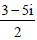• b)• c)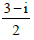• d) None• a) 0
• b) z1 – z2
• c) z1 + z2
• d) 1

#### Question: Universal set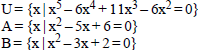What is (A ∩ B)' equal to ?

• a) {1, 3}
• b) {1, 2, 3}
• c) {0, 1, 3}
• d) {0, 1, 2, 3}

• a) 1
• b) 2
• c) 3
• d) 4

#### Question: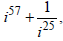when simplified has the value

• a) 0
• b) 2i
• c) – 2i
• d) 2

#### Question: If the roots of x2 + x + a = 0 exceed a then

• a) 2 < a < 3
• b) a > 3
• c) – 3 < a < 3
• d) a < – 2

#### is 4, z ≠ 1, then the locus of the point representing z in the complex plane is

• a) A straight line parallel to x-axis
• b) A straight line equally inclined to axes
• c) A circle with radius 2
• d) a circle with radius
•Answer: a circle with radius#### Question: If α and β are the roots of x2 – x + 1 = 0, then the equation whose roots are α100 and β100 are

• a) x2 – x + 1 = 0
• b) x2 + x – 1 = 0
• c) x2 – x – 1 = 0
• d) x2 + x + 1 = 0

Answer: x2 + x + 1 = 0

• a) π/5
• b) 2π/5
• c) π/10
• d) π/15

#### Question: If x = ω – ω2 –2, then the value of x4 + 3x3 + 2x2– 11x – 6 is

• a) 1
• b) -1
• c) 2
• d) None of these

• a) 1
• b) α2
• c) –1
• d) – α2

#### Question: If the expression x2 – 11x + a and x2 – 14x + 2a must have a common factor and a ≠0, then, the common factor is

• a) (x – 3)
• b) (x – 6)
• c) (x – 8)
• d) None of these

#### Question: If α, β are the roots of the equation ax2 + bx + c = 0, then the roots of the equation ax2 + bx (x + 1)+ c (x + 1)2 = 0 are

• a) α – 1, β– 1
• b) α + 1, β + 1
• c)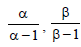• d)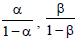#### Question: If a > 0, aεR, z = a + 2i and z | z | – az + 1 = 0 then

• a) Z is always a positive real number
• b) Z is always a negative real number
• c) Z is purely imaginary number
• d) Such a complex z does not exist

Answer: Such a complex z does not exist

#### Question: The roots of the equation x2 – 2 √2 x + 1 = 0 are

• a) Real and different
• b) Imaginary and different
• c) Real and equal
• d) Rational and different

#### Question:  For the equationif the product of roots is zero, then the sum of roots is

• a) 0
• b)• c)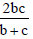• d)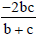#### Question: If arg= arg(z2 ) , then

• a) z2 = kz1–1 (k > 0)
• b) z2 = kz1(k > 0)
• c)• d) None of these

Answer: z2 = kz1–1 (k > 0)

#### and arg(z1 z2) = 0, then

• a) z1 = z2
• b) |z2|2 = z1z2
• c) z1z2 = 1
• d) None of these

#### Question: Let a, b, c € R and ax² + bx + c = 0 has two negative roots, then –

• a) a, b, c are of same sign
• b) a, –b, c are of same sign
• c) a, b, –c are of same sign
• d) a, – c are of same sign

Answer: a, b, c are of same sign

#### Question: If z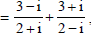then value of arg (zi) is

• a) 0
• b)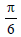• c)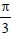• d)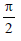#### Question: Value of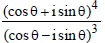is

• a) cos 5θ + i sin 5θ
• b) cos 7θ + i sin 7θ
• c) cos 4θ + i sin 4θ
• d) cosθ + i sinθ

Answer: cos 7θ + i sin 7θ

#### Question: If the roots of the equation x2 + 2ax + b = 0 are real and differ by at most 2m, m ≠ 0 then b lies in the interval

• a) (a2 -m2 , a 2 )
• b) [a2 -m2 , a2 )
• c) (a 2 , a2 + m2 )
• d) None of these

Answer: [a2 -m2 , a2 )

• a) k≤0
• b) k≥0
• c) k≥6
• d) k≤6

• a) 0
• b) 1
• c) 2
• d) 3

• a) p2
• b) 3q
• c) p2 – 2q
• d) p2 – 3q

## More Study Material

### BITSAT Full Syllabus Mathematics Complex Numbers and Quadratic Equations MCQs

We hope students liked the above MCQs for Complex Numbers and Quadratic Equations designed as per the latest syllabus for Full Syllabus Mathematics released by BITSAT. Students of Full Syllabus should download the Multiple Choice Questions and Answers in Pdf format and practice the questions and solutions given in above Full Syllabus Mathematics MCQs Questions on daily basis. All latest MCQs with answers have been developed for Mathematics by referring to the most important and regularly asked topics which the students should learn and practice to get better score in school tests and examinations. Studiestoday is the best portal for Full Syllabus students to get all latest study material free of cost.

### MCQs for Mathematics BITSAT Full Syllabus Complex Numbers and Quadratic Equations

Expert teachers of studiestoday have referred to NCERT book for Full Syllabus Mathematics to develop the Mathematics Full Syllabus MCQs. If you download MCQs with answers for the above chapter daily, you will get higher and better marks in Full Syllabus test and exams in the current year as you will be able to have stronger understanding of all concepts. Daily Multiple Choice Questions practice of Mathematics and its study material will help students to have stronger understanding of all concepts and also make them expert on all critical topics. You can easily download and save all MCQs for Full Syllabus Mathematics also from www.studiestoday.com without paying anything in Pdf format. After solving the questions given in the MCQs which have been developed as per latest course books also refer to the NCERT solutions for Full Syllabus Mathematics designed by our teachers

#### Complex Numbers and Quadratic Equations MCQs Mathematics BITSAT Full Syllabus

All MCQs given above for Full Syllabus Mathematics have been made as per the latest syllabus and books issued for the current academic year. The students of Full Syllabus can refer to the answers which have been also provided by our teachers for all MCQs of Mathematics so that you are able to solve the questions and then compare your answers with the solutions provided by us. We have also provided lot of MCQ questions for Full Syllabus Mathematics so that you can solve questions relating to all topics given in each chapter. All study material for Full Syllabus Mathematics students have been given on studiestoday.

#### Complex Numbers and Quadratic Equations BITSAT Full Syllabus MCQs Mathematics

Regular MCQs practice helps to gain more practice in solving questions to obtain a more comprehensive understanding of Complex Numbers and Quadratic Equations concepts. MCQs play an important role in developing understanding of Complex Numbers and Quadratic Equations in BITSAT Full Syllabus. Students can download and save or print all the MCQs, printable assignments, practice sheets of the above chapter in Full Syllabus Mathematics in Pdf format from studiestoday. You can print or read them online on your computer or mobile or any other device. After solving these you should also refer to Full Syllabus Mathematics MCQ Test for the same chapter

#### BITSAT MCQs Mathematics Full Syllabus Complex Numbers and Quadratic Equations

BITSAT Full Syllabus Mathematics best textbooks have been used for writing the problems given in the above MCQs. If you have tests coming up then you should revise all concepts relating to Complex Numbers and Quadratic Equations and then take out print of the above MCQs and attempt all problems. We have also provided a lot of other MCQs for Full Syllabus Mathematics which you can use to further make yourself better in Mathematics

You can download the BITSAT MCQs for Full Syllabus Mathematics Complex Numbers and Quadratic Equations for latest session from StudiesToday.com

Yes, you can click on the links above and download topic wise MCQs Questions PDFs for Complex Numbers and Quadratic Equations Full Syllabus for Mathematics

Are the Full Syllabus Mathematics Complex Numbers and Quadratic Equations MCQs available for the latest session

Yes, the MCQs issued by BITSAT for Full Syllabus Mathematics Complex Numbers and Quadratic Equations have been made available here for latest academic session